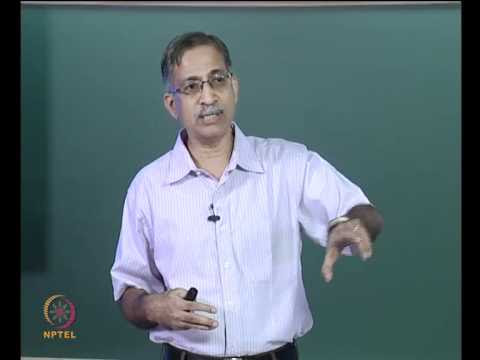This course mainly deals with matrix analysis of structures.
It begins with a review of the basic concepts of structural analysis and matrix algebra, and shows how the latter provides an excellent mathematical framework for the former.
This is followed by detailed descriptions, and demonstrations through many examples, of how matrix methods can be applied to linear static analysis of skeletal structures (plane and space trusses; beams and grids; plane and space frames) by the stiffness method, and also the flexibility method.
Also, it is shown how simple structures can be conveniently solved using a reduced stiffness formulation, involving far less computational effort.
Finally, the analysis of elastic instability and second-order response is discussed.
The main objective is to enable the student to have a good grasp of all the fundamental issues in these advanced topics in structural analysis, besides enjoying the learning process, and developing analytical and intuitive skills.
This course is also expected to enable a good understanding of how standard software packages (routinely used for frame analysis in design offices) operate.
Moreover, the student will be well prepared to explore and understand further topics like Finite Element Analysis.
 Sl. No. Topic No. of Hours 1 Review of basic concepts in structural analysis: structure (structural elements, joints and supports, stability, rigidity and static indeterminacy, kinematic indeterminacy); loads (direct actions, indirect loading); response (equilibrium, compatibility, force-displacement relations); levels of analysis; analysis of statically determinate structures (trusses, beams, frames); applications of principle of virtual work and displacement-based and force-basedenergy principles; deriving stiffness and flexibility coefficients. 2 2 Review of analysis of indeterminate structures: Force methods: Statically indeterminate structures (method of consistent deformations; theorem of least work). Displacement Methods: Kinematically indeterminate structures (slope-deflection method; moment distribution method). 4 3 Matrix concepts and Matrix analysis of structures: Matrix; vector; basic matrix operations; rank; solution of linear simultaneous equations; eigenvalues and eigenvectors. Introduction; coordinate systems; displacement and force transformation matrices; Contra-gradient principle; element and structure stiffness matrices; Element and structure flexibility matrices; equivalent joint loads; stiffness and flexibility approaches. 5 4 Matrix analysis of structures with axial elements: Introduction: Axial stiffness and flexibility; stiffness matrices for an axial element (two dof), plane truss element (four dof) and space truss element (six dof); One-dimensional axial structures: Analysis by conventional stiffness method (two dof per element) and reduced element stiffness method (single dof); Analysis by flexibility method; Plane trusses: Analysis by conventional stiffness method (four dof per element) and reduced element stiffness method (single dof); Analysis by flexibility method; Space trusses: Analysis by conventional stiffness method (six dof per element) and reduced element stiffness method (single dof). 8 5 Matrix analysis of beams and grids: Conventional stiffness method for beams: Beam element stiffness (four dof); generation of stiffness matrix for continuous beam; dealing with internal hinges, hinged and guided-fixed end supports; accounting for shear deformations; Reduced stiffness method for beams: Beam element stiffness (two dof); dealing with moment releases, hinged and guided-fixed end supports; Flexibility method for fixed and continuous beams: Force transformation matrix; element flexibility matrix; solution procedure (including support movements); Stiffness method for grids: Introduction; torsional stiffness of grid element and advantage of torsion release; analysis by conventional stiffness method using grid element with six dof; analysis by reduced stiffness method (three dof per element); 10 6 Matrix analysis of plane and space frames: Conventional stiffness method for plane frames: Element stiffness (six dof); generation of structure stiffness matrix and solution procedure; dealing with internal hinges and various end conditions; Reduced stiffness method for plane frames: Element stiffness (three dof); ignoring axial deformations; dealing with moment releases, hinged and guided-fixed end supports; Flexibility method for plane frames: Force transformation matrix; element flexibility matrix; solution procedure (including support movements); Ignoring axial deformations; Stiffness method for space frames: Introduction; element stiffness matrix of space frame element with 12 dof and 6 dof; coordinate transformations; analysis by reduced stiffness method (six dof per element); 7 7 Analysis of elastic instability and second-order effects: Effects of axial force on flexural stiffness: Review of buckling of ideal columns; flexural behaviour and stiffness measures for beam-columns - braced and unbraced, under axial compression; Solution by slope deflection method: Slope deflection equations for prismatic beam columns using stability functions; modifications for pinned and guided-fixed-end conditions; fixed-end moments in beam-columns; Solution by matrix method: Stiffness matrix for prismatic beam-column element; estimation of critical elastic buckling loads; second-order analysis; 7 Total 43